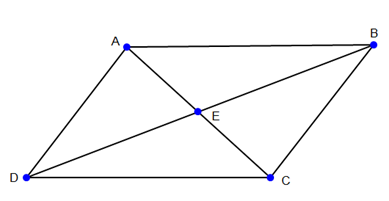• The learning process is entertaining and exciting
• The solved tasks turn into coordinates
• Along with your schoolmates and friends, go on an adventure, searching for hideouts
• E-mail:

# 2445.3. Parallelogram, elements. Properties and signs.

#### The parallelogram ABCD is given. It's diagonals cross in a point E.∢AED = 45°, ∢BDC=20°, ∢ACB =85°.
∢ABC=

#### Next task: 1516. Parallelogram, elements. Properties and signs.

8
Subject
Geometry
Theme
Parallelogram.
Subtheme
Parallelogram, elements. Properties and signs.

#### Solve tasks to find hidden Geo point coordinates

1. Algebra: Viet theorem.
2. Chemistry: Acids and bases. Indicators.
3. Geometry: Rhombus, its properties and signs.
4. Chemistry: Waters chemical properties and formation.
5. Algebra: Irrational numbers.
6. Geometry: Squar, properties and signs.
7. Algebra: Viet theorem.
8. Physics: Sounds characteristics.
9. Physics: Heat expanding.
10. Algebra: Fraction amplification and abbreviation.